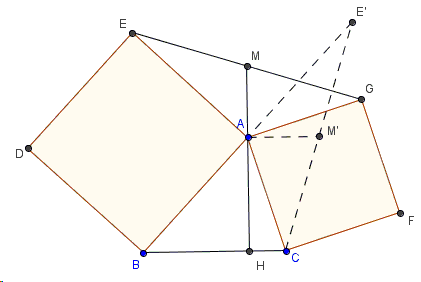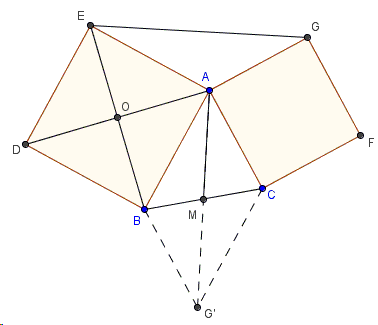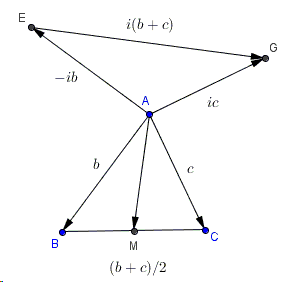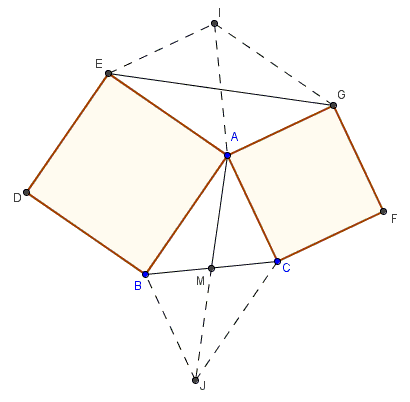# Properties of Flank Triangles

Bottema's configurationof two squares that share a vertex is naturally embedded into Vecten's configuration of three squares erected on the sides of a triangle. The latter generalizes the first of Euclid's proofs of the Pythagorean theorem, so it rightfully refers to the Bride's Chair.

In the discussion of Vecten's configuration, we proved inter alia two properties of flank triangles that are a part of Bottema's configuration.

1. Continuation of a median (altitude) from the common vertex in one flank triangle form the altitude (median) in the other.

2. The base opposite the common vertex in one flank triangle is twice as long as the median from the common vertex in the other.With a reference to the diagram,

1. If $AM$ is a median in $\triangle ABC$, $AH$ is an altitude in $\triangle AEG$, then $M$, $A$, $H$ are collinear.

2. $EG = 2\cdot AM$.

I'll give five proofs of these properties, three using rotation transform, one with complex variables, and one with an extra construction that has the virtue of revealing an additional property of Bottema's configuration. All five proofs qualify as proofs without words, however, I placed a dynamic demonstration of each on a separate page.

### Proof 1### Proof 2### Proof 3### Proof 4### Proof 5### Bottema's Theorem